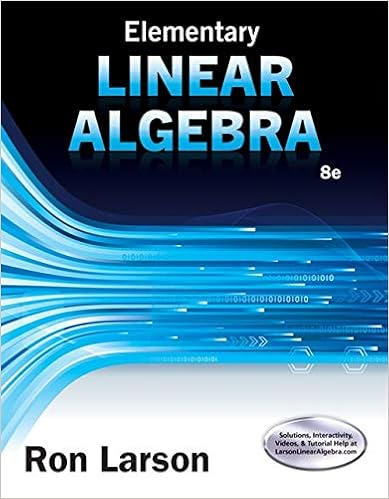Final Exam - Math 2090 Final Exam Spring 2011 Britt Work...

• Notes
• 2
• 100% (7) 7 out of 7 people found this document helpful

This preview shows page 1 - 2 out of 2 pages.

We have textbook solutions for you!
The document you are viewing contains questions related to this textbook.The document you are viewing contains questions related to this textbook.
Chapter 7 / Exercise 20
Elementary Linear Algebra
LarsonExpert Verified
Math 2090 Final Exam Spring 2011 Britt Work the problems in order on the fronts of your paper. Show your work. l[ ] 1. Compute the Laplace Inverse L- ') s+2 . s~ + y" y' - 2y -= 6e- , 2. Detennine L[ (t -1/ u 4 (t) 3. Solve the following initial value problem by using Laplace Transfonns yeO) = 0, y'(O) '> (''-LA - , ! ~ 4. Solve the following differentIal equations -3x a) y' -2ysinx b)y,+.ly=e\ x>O c) Y " - X 8 d) y"-4y Hint: Use Variation of Parameters 5. Let A be a non-singular n x n matrix. List three other statements which are equivalent to this fact. 6. Solve the following linear system using Gauss-Jordan reduction. 3x 2y z=4 x+2y z=6 2x+ y+z 7. Compute the inverse ofthe matrix r ~ !i by using row reduction. No other method will , get credit. 8. Detennine if the following are vector spaces. EXPLAIN YOUR REASONING if you are claiming they are not a vector space. 2s + 2 l J = 1 Y = 8 e =9 J A) The set of2x2 matrices with a 0 in position 2,2 with operations defined in the standard manner. B) The set of all increasing functions on JR with operations defined in the usual manner.
We have textbook solutions for you!
The document you are viewing contains questions related to this textbook.The document you are viewing contains questions related to this textbook.
Chapter 7 / Exercise 20
Elementary Linear Algebra
LarsonExpert Verified
•••